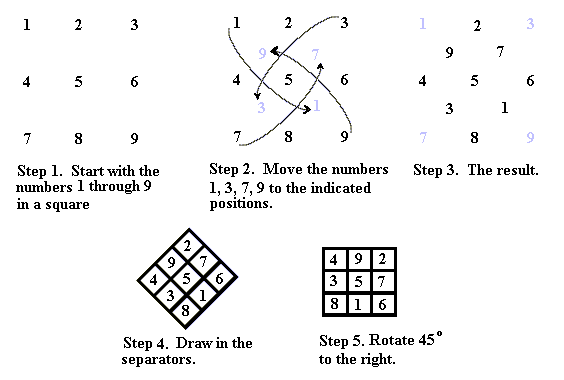Magic Squares of Odd Orders   Let us now turn our attention to magic squares of odd order; that is, magic squares that have an odd number of rows and columns. At the beginning, we saw the 33 lo-shu magic square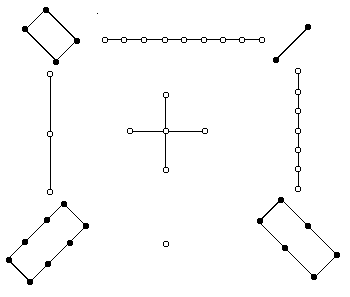and its modern representation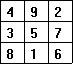Let us now look at how one can generate magic squares of any odd order (3, 5, 7, 9, ..., 2n + 1, ...). While as an envoy of Louis XIV to Siam in 1687-1688, De la Loubère learned a simple way of generating magic squares of odd orders. This method, some call the De la Loubère method and others call it the Siam method, will work to generate a magic square of any odd order. We will generate a 7th-order magic square as an example and the reader may see how 3rd- and 5th-order squares can be generated. To create the 77 magic square we start with a 77 matrix and we put extra cells along the top and right-hand side. We then either shade or x-out the cell in the upper right-hand corner. Our beginning square should look like this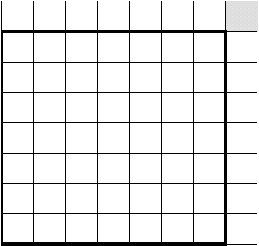. The method that De la Loubère brought back from Siam was as follows. In the center square of the top row place the number 1. The algorithm we will use is to move up one and to the right one to place the next number. If we go out of our square to place the number, we consider the extra cells above the top row to be the same as the bottom row of our square and the extra cells to the right of our square to be the same as the cells of the left-hand column of our square. So, since the number 2 would be placed in a cell above our square, we move it straight down to the corresponding cell in the bottom row. We then proceed with the up one, to the right one scheme. This is fine until we place the number 5 outside our square in the extra cells to the right. We therefore move the 5 directly across to the corresponding cell on the left side of our square.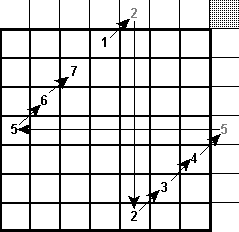We continue on until we place the 7. We now have a problem. The cell that is up one and to the right one is occupied by the number 1. In this case, we add a new rule to our algorithm. That is, if we reach a cell that is already occupied we drop down to the empty cell directly below the last number entered. Therefore we place the number 8 directly below the cell containing the number 7.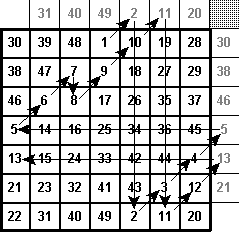We continue this process until we place the number 28 in the upper right-hand cell of our square. We now see the reason for shading the one outside cell. It does not correspond to any cell in our square, so we consider it the same as an occupied cell and drop straight down one cell for the next number. Below is the finished product.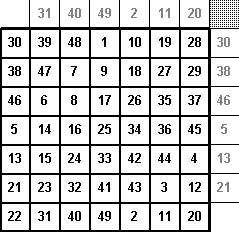Being an odd ordered magic square, the lo-shu can be constructed using the above method and flipping the square through a horizontal axis. Another way to construct the lo-shu magic square is given in the following illustration.